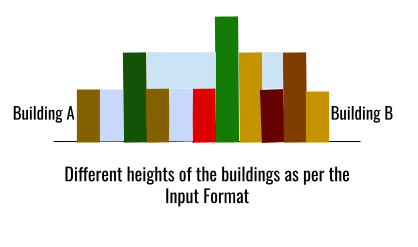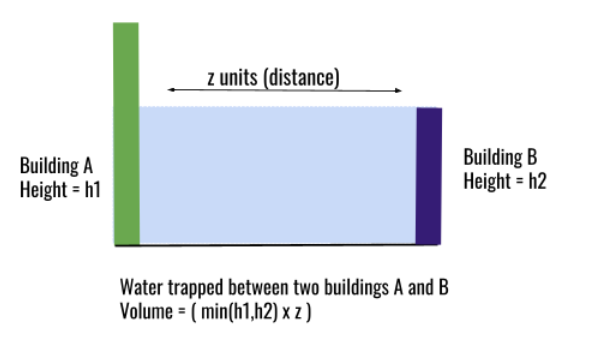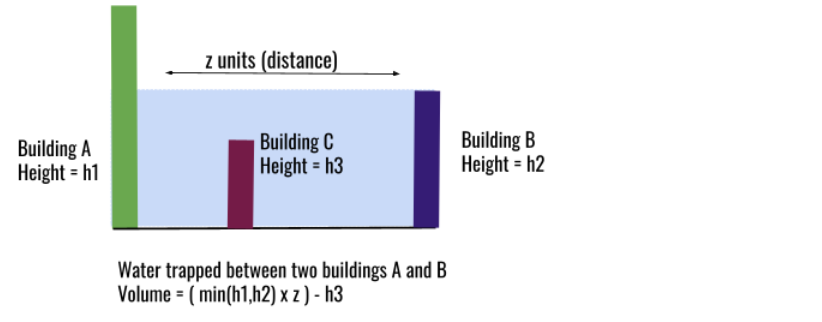# Trapping Rainwater

## Introduction

Flatland experienced the heaviest rainfall last night. All the squares came to the rescue. But they need to know the volume of trapped rainwater between the buildings to remove it. As every figure in Flatland is in 2-D, the width of the buildings can be considered as unity(1).

## Problem Statement

An elevation map of Flatland is given as an array. Every element represents the height of the building. The width of the buildings can be considered 1. Find the volume of trapped rainwater between these buildings.

Input Format

``````Size of the array: 12
Enter the elements:
0    1    0    2    1    0    1    3    2    1    2    1``````

``Volume of trapped rainwater: 6``

Input Illustration### Understanding the Problem

What will be the volume of trapped rainwater stored between two outer buildings A and B, if all the other buildings present in between them are removed?

After removing all the buildings except the extremes, the structure looks like the figure shown below.What will be the volume of trapped rainwater if there is another building C between building A and B? Look at the figure given below for illustration:It is not difficult to calculate the new volume of trapped rainwater. The initial volume is calculated by min(h1,h2) x z . The new volume can be calculated by removing the volume occupied by building C (h3).

Also, if you notice, the water level is not changed as the height h3 is less than height h1 and h2.

So, when does the water level change? Let's add another building D with height h4. Consider the figure given below:

Through this series of different scenarios, we can understand that the water level for a specific building changes with the change in maximum heights of buildings present on the right and left.

From here on, the building with maximum height on the right side will be called right_max. Similarly, a building with maximum height on the left side will be called left_max. Please note that the values of right_max and left_max change with every building. So, to calculate the volume of the trapped rainwater, we need to find min(right-max, left-max) for every building(array element).

Thus, we have converted a highly complex problem to a simpler one. Isn’t it exciting?

Let’s look at the various methods to solve our simple problem.

### Method 1: Brute Force

The simplest way to find the right_max and left_max for every element is a linear comparison with all other elements. Compare every element on the right side with right_max. The maximum of both is the new right_max. Similarly, left_max is found by comparing left_max to elements present on the left side of the current array element.

Algorithm

• Initialize total_volume = 0.
• Set size = size of heights.
• Iterate through the heights from 0 to size - 1.
• Initialize left_max = 0.
• Iterate through the heights from 0 to current element.
• Set left_max = max(left_maxcurrent element).
• Initialize right_max = 0.
• Iterate through the heights from current element to size.
• Set right_max = max(right_maxcurrent element).
• Add min(left_maxright_max) - current element to total_volume.
• Return total_volume.

Code for Reference

``````#include <bits/stdc++.h>
using namespace std;

int rainWaterVol(vector<int> heights) {
int total_volume = 0;
int size = heights.size();

for(int current = 1; current < size - 1; current++) {

// Find left_max for current element.
int left_max = heights[current];
for(int left = 0; left < current; left++) {
left_max = max(left_max, heights[left]);
}

// Find right_max for current element.
int right_max = heights[current];
for(int right = current + 1; right < size; right++){
right_max = max(right_max, heights[right]);
}

// Adding trapped rainwater volume to the total volume.
total_volume += min(left_max, right_max) - heights[current];
}

}

int main() {
int size;
cout << "Size of the array: ";
cin >>  size;

vector<int> heights(size);
cout << "Enter the elements:\n";
for (int i = 0; i < size; i++) {
cin >> heights[i];
}

cout << "Volume of trapped rainwater: " << rainWaterVol(heights);
}``````

Input Format

``````Size of the array: 12
Enter the elements:
0    1    0    2    1    0    1    3    2    1    2    1
``````

Output Format

``Volume of trapped rainwater: 6``

Complexity Analysis

Time Complexity: The code uses two nested linear loops to find the right_max and left_max. So, the time complexity for this method is O(N2)

Space Complexity: Constant space is used. So, the space required is independent of the size of the input given. Space complexity is O(1).

### Method 2: Dynamic Programming

For every element, we need to find right_max and left_max.Avoid iterating the right and left sides for every element. Instead, we can create two lookups for right_max and left_maxright_max_array and left_max_array.

So, the algorithm for this new method can be modified as given below.

Algorithm

• Initialize total_volume = 0.
• Set size = size of heights.
• Declare vectors right_max_array and left_max_array of lengths equal to size.
• Set right_max_array = heights.
• Set left_max_array[size - 1] = heights[size - 1].
• Iterate through the heights from 1 to size for left_max_array.
• Set left_max_array[i] = max(left_max_array[i - 1], heights[i]).
• Iterate through the heights from size - 2 to 0 for right_max_array.
• Set right_max_array[i] = max(rightt_max_array[i + 1], heights[i]).
• Iterate through the heights from 1 to size -1 to calculate the total_volume.
• Add max(right_max_array[i], left_max_array[i]) - height[i] to total_volume.

Code for Reference

``````#include <bits/stdc++.h>
using namespace std;

int rainWaterVol(vector<int> heights) {
int total_volume = 0, size = heights.size();

// Edge case: if the input is empty
if(size == 0) {
return 0;
}

// Declaring lookup tables.
vector<int> right_max_array(size), left_max_array(size);
left_max_array = heights;
right_max_array = heights[size-1];

// Filling right_max_array lookup table.
for(int current = 1; current < size; current++) {
left_max_array[current] = max(left_max_array[current-1], heights[current]);
}

// Filling left_max_array lookup table.
for(int current = size - 2; current >= 0; current--) {
right_max_array[current] = max(right_max_array[current+1], heights[current]);
}

// Calculating total_volume using lookups.
for(int current = 1; current < size-1; current++) {
total_volume += min(left_max_array[current], right_max_array[current])- heights[current];
}
}

int main() {
int size;
cout << "Size of the array: ";
cin >>  size;

vector<int> heights(size);
cout << "Enter the elements:\n";
for (int i = 0; i < size; i++) {
cin >> heights[i];
}

cout << "Volume of trapped rainwater: " << rainWaterVol(heights);
}``````

Input Format

``````Size of the array: 12
Enter the elements:
0    1    0    2    1    0    1    3    2    1    2    1``````

Output Format

``Volume of trapped rainwater: 6``

Complexity Analysis

Time Complexity: Creating lookups is a linear-time operation. Also, calculating total_volume using the lookups requires a one-time iteration through the given input. So, the time complexity is O(N).

Space Complexity: Extra space is required to create lookups. The size of lookups depends linearly on the size of the input. So, the space complexity is O(N).

### Method 3: Monotonic Stack

So far we have been calculating the volume of trapped rainwater for every building. For this, we needed to find the right_max and left_max.

How is this avoided in the stack? In a stack, every time there’s an increase in height, the volume of the trapped water between new increase(current height) and old increase(top of the stack) is calculated. So, for heights smaller than the top of the stack, values are pushed in the stack. When heights larger than the top of the stack are encountered, we can assume that water is trapped between the index present at the top of the stack and the current index. Refer to the illustration given below for a better understanding.

Please note that the values stored in the stack are not the heights of buildings but indexes of these heights.

Algorithm

• Create an empty stack currentStack.
• Loop current from 0 to size of heights array.
• While currentStack is not empty and height[current] > height[top element of currentStack]:
• Initialize top height[top element of currentStack].
• Pop the top element of the stack.
• Initialise distance current - new top element of currentStack - 1.
• Initialise height_difference = min(height[current], height[new top element in currentStack]) - heights[top].
• Set volume distance height_difference.
• Push current to the stack.
• Return total_volume.

Code for Reference

``````#include <bits/stdc++.h>
using namespace std;

int rainWaterVol(vector<int> heights) {
int total_volume = 0, size = heights.size();
stack<int> currentStack;

for(int current = 0; current < size; current++) {

/* Calculating the volume of rainwater trapped water trapped
between the index present at the top of the stack
and the current index. */
while(!currentStack.empty() && heights[current] > heights[currentStack.top()]) {

int top = currentStack.top();
currentStack.pop();

if(currentStack.empty())
break;

int distance = current - currentStack.top() -1;
int height_difference = min(heights[current],           heights[currentStack.top()]) - heights[top];

int volume = distance * height_difference;
total_volume += volume;

}

/* Pushing the current index if it is smaller than
the index present on the top of the stack.*/
currentStack.push(current);
}

}

int main() {
int size;
cout << "Size of the array: ";
cin >>  size;

vector<int> heights(size);
cout << "Enter the elements:\n";
for (int i = 0; i < size; i++) {
cin >> heights[i];
}

cout << "Volume of trapped rainwater: " << rainWaterVol(heights);
}``````

Input Format

``````Size of the array: 12
Enter the elements:
0    1    0    2    1    0    1    3    2    1    2    1``````

Output Format

``Volume of trapped rainwater: 6``

Complexity Analysis

Time Complexity: Every element is pushed exactly once in a stack. So, the time complexity is O(n).

Space Complexity: In the worst-case scenario, the stack may need to store all the indexes of the heights vector. So, the space complexity is O(n).

### Method 4: Two Pointers Technique

Do you remember how we calculated the volume of trapped rainwater in methods 1 and 2? We used the formula min(right-max, left-max) - heights[current]. This means the calculation requires a minimum of the extremes to calculate the volume. This is our basis for a two-pointers approach.

It’s evident that right_max and left_max will be found on the right and left sides of a building, respectively. We use left pointer to keep track of elements for which min(right-max, left-max) =current left-max . Similarly, the right pointer is used so that we don’t have to calculate min(right-max, left-max) for every building. So, left and right are the two pointers of our two-pointers approach. Please note, the left will always be smaller than the right

Refer to the following algorithm for better understanding.

Algorithm

• Initialize left = 0 (Index of current where min(right-max, left-max) =left-max ).
• Initialize right heights.size() - 1(Index of current where min(right-max, left-max) =right-max).
• Set right_max and left_max equal to 0.
• While left right, do:
• If heights[left] heights[right], then:
• Set left_max = max(left_maxheights[left]).
• Add left_max heights[left] to volume.
• Increment left.
• Else:
• Set right_max = max(right_maxheights[right]).
• Add right_max heights[right] to volume.
• Decrement right.

Code for Reference

``````#include <bits/stdc++.h>
using namespace std;

int rainWaterVol(vector<int> heights) {
int total_volume = 0;

// left and right pointer to track current left_max and right_max.
int left = 0 , right = heights.size() - 1;
int right_max = 0, left_max = 0;

while(left < right) {

/* If current left_max is less than current right_max,
water level depends on left_max.*/
if(heights[left] < heights[right]) {
left_max = max(heights[left], left_max);
total_volume += left_max - heights[left];
left++;
}
else {

/* If current right_max is less than current left_max.
Water level depends on right_max.*/
right_max = max(heights[right], right_max);
total_volume += right_max - heights[right];
right--;
}

}
}

int main() {
int size;
cout << "Size of the array: ";
cin >>  size;

vector<int> heights(size);
cout << "Enter the elements:\n";
for (int i = 0; i < size; i++) {
cin >> heights[i];
}

cout << "Volume of trapped rainwater: " << rainWaterVol(heights);
}``````

Input Format

``````Size of the array: 12
Enter the elements:
0    1    0    2    1    0    1    3    2    1    2    1``````

Output Format

``Volume of trapped rainwater: 6``

Complexity Analysis

Time Complexity: Both the pointers start the iteration from the extremes and iterate till they meet. So, a linear loop is completed. Time complexity is O(N).

Space Complexity: Constant space is used. So, the space complexity is O(1).

## Key Takeaways

There’s usually more than one approach to a solution. Yes, it feels sluggish to solve the same problem again and again. But, we should always try to look for more ways to solve a problem. After all, it’s an excellent way to practice more than one algorithm or technique from a single problem

Try to memoize the code if some value is computed again and again. If the memoization is in a particular order, go for the monotonic stacks. You can practice memoization and many more cool techniques using our free practice platform CodeStudio. So, keep practicing. That’s what good coders do.

Happy Coding!

Pranav Gautam